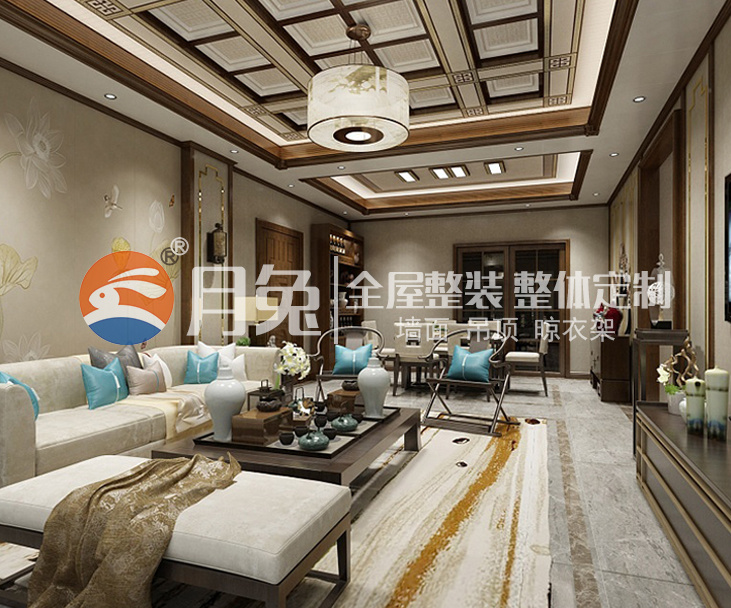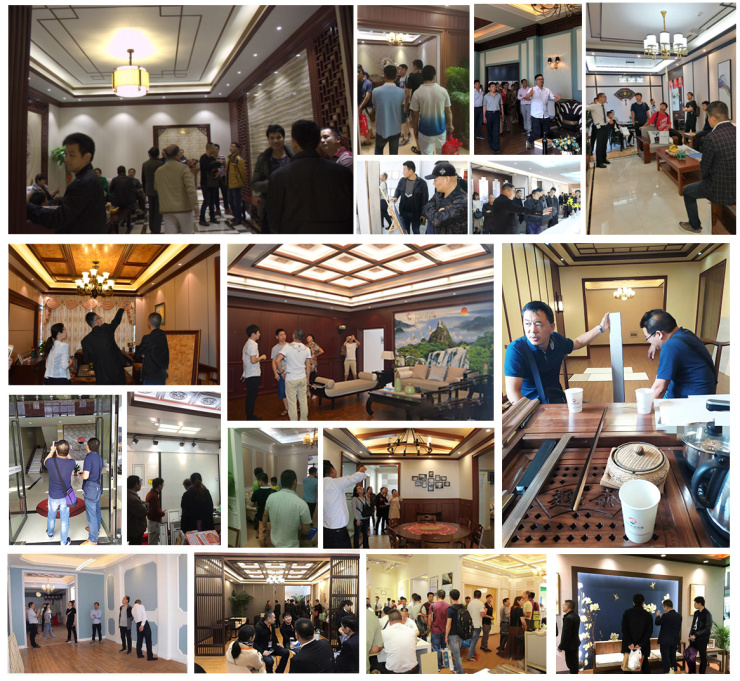|

# 西安集成墙面 集成墙面市场利润多大?

每次我们都是介绍的竹木纤维集成墙面，最近有不少西安的客户咨询月兔客服有关于铝合金集成墙面的相关问题，那么本期文章我们就来看看有关于西安铝合金集成墙面相关知识，如何鉴定区别铝合金集成墙面质量的好坏!

我们知道铝复合集成墙面的结构是由表面装饰膜、铝板、聚氨酯发泡层、铝箔组成。所以在拿到一块铝复合集成墙面的时候，关注的重点绝不应该仅仅是表面的花色好不好看，应该层层检验，在条件允许的情况下。西安集成墙面鉴定：首先是铝合金层

看色度:优质的铝合金色泽应一致,如色差明显,即不宜选购。一般正常铝合金型材截面颜色为银白色,质地均匀,如果颜色暗黑,可能为回收铝或者废铝回炉锻造而成。好的铝合金切口亮丽，光泽度好。差的铝合金切口处黯淡，没有光泽。

看平整度:用手细细感受，铝合金表面应无凹陷或鼓出。正规厂家加工出来的墙板表面平整光亮。

看厚度：市面上铝复合集成墙面的厚度一般在1.0-1.5公分，而表面铝板的厚度也是有差异的，目前了解到的厚度一般在0.5-0.6公分之间。所以铝板的厚度要综合整个墙板的厚度来综合考虑。铝板太薄的话，安全性要低一点。

西安集成墙面鉴定：看聚氨酯发泡层

我们之前讲过聚氨酯发泡很大程度上决定了墙板的保温、防潮、防火等功能。有资料显示，聚氨酯层的厚度在北方通常使用为55mm以上，在南方在45mm以上就足够了。太薄，无法实现较好的保温效果。

第二，聚氨酯层不能光看厚度，最主要的是要看发泡工艺。发泡后的密度为3.8kg/m³——这个是黄金密度比，在这个密度的聚氨酯是保温性能最好的;通常想要保证发泡工艺的唯一办法就是通过恒温发泡环境、高压抢、定量进料控制来实现，手工操作是无法完成的，需要依靠微电脑控制。

第三，保温效果好不好，还要看聚氨酯发泡后的闭孔率，也就是里面那些小泡泡是不是都是完整的泡泡，有多少是碎的，闭孔率在90%以上才是好的聚氨酯。

有些无法用肉眼看的出来!不过可以感受下聚氨酯的厚度，硬度以及是否容易损坏，来对产品做一个粗略的估计。

铝箔：为了为了加强产品的抗腐性所设计，大大地加强了产品的使用寿命。 这是一个娇弱的东西，过多不详细叙述。可以看看墙板上铝箔的平整度如何，小小的细节可是能暴露厂家的生产标准的哟。

西安集成墙面鉴定：看膜的材质

产品的色彩效果主要是通过表面的膜来体现的，那么所用的膜的好坏也决定着产品的好坏。千万不要小看这简简单单的一层膜，它也分好坏的，好的膜厚度适中，纹底效果逼真，有立体感和凹凸感而且无异味;

反之一些差的膜可能是用废旧的塑料或其他劣质材料做的，比较薄，容易透底。在选择的时候可以先看看质感、效果，尝试下是否容易破坏。闻闻味道也是一种考察方法。

核心：膜是怎么结合在铝合金表面的

还有一个核心的关键，就是膜是如何覆在铝合金表面的，这个对最终的产品的使用寿命和是否达到真环保，也起着决定性的作用。

目前我们了解到的方法有两种，高温覆膜法和冷胶法。

高温覆膜使铝合金和膜在300度左右的高温下直接粘合在一起，能实现无甲醛，贴合平整的优质效果。

冷胶则是在膜体上涂胶水然后和铝合金板粘合在一起。至于是否环保以及使用寿命就要看厂家使用的胶水品质以及粘合工艺，劣质胶水不仅会挥发有害成分且使用效果无法担保。

专家表示：开集成墙面店的利润主要是看自己所选择的品牌，所做的产品、所在的市场而决定的，品牌越好，产品质量越高，市场越好，所产生的利润也就越高，但是，普遍的利润在三分之一左右。

可以说开集成墙面店是属于一个低投入、高回报的行业，所以，现在开集成墙面是能够赚到钱的。但是，在这里有必要提醒大家一点的就是，开集成墙面店选择一个好的品牌是非常重要的。

但是，作为集成墙面加盟商们，要如何使得利润最大化?又有什么办法能够让企业快速扩大利润收入呢?

及时沟通高效工作

集成墙面厂家和加盟代理商合作的过程中，难免会因为沟通不畅造成误会，所以遇到问题要及时沟通，这样才能让厂家知道集成墙面加盟代理商真正的想法，了解市场当前的现状，根据实际情况制定解决方案，帮助加盟商快速打开市场。

大品牌有保障

对于很多人来说，都是希望能够用最少的投入换取最大的回报，因此很多加盟商在选择集成墙面品牌的时候，就尽可能寻找一些投资小的品牌，这样一来，成本虽然降低，但是产品的质量却没有保证，一旦出现质量问题，对于代理商来说简直是灾难。

许多的品牌厂商考虑到集成墙面加盟的一些实际困难，因此在进行产品招商的时候，也推出了一系列优惠政策，包括零加盟费+零保证金的双免政策，让加盟商可以不花一分钱就能获得大品牌的代理资格。这样一来，产品质量有了保证，加盟商也可以更加有信心去做好市场宣传和销售工作。

别出心裁吸引顾客

除了要有知名的品牌作为货源渠道之外，集成墙面加盟代理商还要自己研究新的推广宣传方法来吸引更多的消费者。特别是对于插进去吗这种新型的产品，单纯的讲解产品性能和优点已经不能满足消费者的需要，消费者要考虑到产品的样式与其他类的搭配程度等，这样才能让顾客看到产品的设计理念与风格以及产品的材质与质量。尊重客户提高服务

服务行业要把消费者放在第一位，所以不管是要购买产品的或是了解产品的顾客，都要尊重他们，微笑迎接，耐心讲解，给消费者留下一个良好的印象。同时，也要加强自身业务能力培训和售后服务质量，一旦出现问题，要快速帮助消费者解决问题，树立良好的服务形象。

`声明：本文由入驻焦点开放平台的作者撰写，除焦点官方账号外，观点仅代表作者本人，不代表焦点立场错误信息举报电话： 400-099-0099，邮箱：jubao@vip.sohu.com，或点此进行意见反馈，或点此进行举报投诉。`A B C D E F G H J K L M N P Q R S T W X Y Z
A - B - C - D - E
• A
• 鞍山
• 安庆
• 安阳
• 安顺
• 安康
• 澳门
• B
• 北京
• 保定
• 包头
• 巴彦淖尔
• 本溪
• 蚌埠
• 亳州
• 滨州
• 北海
• 百色
• 巴中
• 毕节
• 保山
• 宝鸡
• 白银
• 巴州
• C
• 承德
• 沧州
• 长治
• 赤峰
• 朝阳
• 长春
• 常州
• 滁州
• 池州
• 长沙
• 常德
• 郴州
• 潮州
• 崇左
• 重庆
• 成都
• 楚雄
• 昌都
• 慈溪
• 常熟
• D
• 大同
• 大连
• 丹东
• 大庆
• 东营
• 德州
• 东莞
• 德阳
• 达州
• 大理
• 德宏
• 定西
• 儋州
• 东平
• E
• 鄂尔多斯
• 鄂州
• 恩施
F - G - H - I - J
• F
• 抚顺
• 阜新
• 阜阳
• 福州
• 抚州
• 佛山
• 防城港
• G
• 赣州
• 广州
• 桂林
• 贵港
• 广元
• 广安
• 贵阳
• 固原
• H
• 邯郸
• 衡水
• 呼和浩特
• 呼伦贝尔
• 葫芦岛
• 哈尔滨
• 黑河
• 淮安
• 杭州
• 湖州
• 合肥
• 淮南
• 淮北
• 黄山
• 菏泽
• 鹤壁
• 黄石
• 黄冈
• 衡阳
• 怀化
• 惠州
• 河源
• 贺州
• 河池
• 海口
• 红河
• 汉中
• 海东
• 怀来
• I
• J
• 晋中
• 锦州
• 吉林
• 鸡西
• 佳木斯
• 嘉兴
• 金华
• 景德镇
• 九江
• 吉安
• 济南
• 济宁
• 焦作
• 荆门
• 荆州
• 江门
• 揭阳
• 金昌
• 酒泉
• 嘉峪关
K - L - M - N - P
• K
• 开封
• 昆明
• 昆山
• L
• 廊坊
• 临汾
• 辽阳
• 连云港
• 丽水
• 六安
• 龙岩
• 莱芜
• 临沂
• 聊城
• 洛阳
• 漯河
• 娄底
• 柳州
• 来宾
• 泸州
• 乐山
• 六盘水
• 丽江
• 临沧
• 拉萨
• 林芝
• 兰州
• 陇南
• M
• 牡丹江
• 马鞍山
• 茂名
• 梅州
• 绵阳
• 眉山
• N
• 南京
• 南通
• 宁波
• 南平
• 宁德
• 南昌
• 南阳
• 南宁
• 内江
• 南充
• P
• 盘锦
• 莆田
• 平顶山
• 濮阳
• 攀枝花
• 普洱
• 平凉
Q - R - S - T - W
• Q
• 秦皇岛
• 齐齐哈尔
• 衢州
• 泉州
• 青岛
• 清远
• 钦州
• 黔南
• 曲靖
• 庆阳
• R
• 日照
• 日喀则
• S
• 石家庄
• 沈阳
• 双鸭山
• 绥化
• 上海
• 苏州
• 宿迁
• 绍兴
• 宿州
• 三明
• 上饶
• 三门峡
• 商丘
• 十堰
• 随州
• 邵阳
• 韶关
• 深圳
• 汕头
• 汕尾
• 三亚
• 三沙
• 遂宁
• 山南
• 商洛
• 石嘴山
• T
• 天津
• 唐山
• 太原
• 通辽
• 铁岭
• 泰州
• 台州
• 铜陵
• 泰安
• 铜仁
• 铜川
• 天水
• 天门
• W
• 乌海
• 乌兰察布
• 无锡
• 温州
• 芜湖
• 潍坊
• 威海
• 武汉
• 梧州
• 渭南
• 武威
• 吴忠
• 乌鲁木齐
X - Y - Z
• X
• 邢台
• 徐州
• 宣城
• 厦门
• 新乡
• 许昌
• 信阳
• 襄阳
• 孝感
• 咸宁
• 湘潭
• 湘西
• 西双版纳
• 西安
• 咸阳
• 西宁
• 仙桃
• 西昌
• Y
• 运城
• 营口
• 盐城
• 扬州
• 鹰潭
• 宜春
• 烟台
• 宜昌
• 岳阳
• 益阳
• 永州
• 阳江
• 云浮
• 玉林
• 宜宾
• 雅安
• 玉溪
• 延安
• 榆林
• 银川
• Z
• 张家口
• 镇江
• 舟山
• 漳州
• 淄博
• 枣庄
• 郑州
• 周口
• 驻马店
• 株洲
• 张家界
• 珠海
• 湛江
• 肇庆
• 中山
• 自贡
• 资阳
• 遵义
• 昭通
• 张掖
• 中卫

1室1厅1厨1卫1阳台

1
2
3
4
5

0
1
2

1

1

0
1
2
3报名成功，资料已提交审核A B C D E F G H J K L M N P Q R S T W X Y Z
A - B - C - D - E
• A
• 鞍山
• 安庆
• 安阳
• 安顺
• 安康
• 澳门
• B
• 北京
• 保定
• 包头
• 巴彦淖尔
• 本溪
• 蚌埠
• 亳州
• 滨州
• 北海
• 百色
• 巴中
• 毕节
• 保山
• 宝鸡
• 白银
• 巴州
• C
• 承德
• 沧州
• 长治
• 赤峰
• 朝阳
• 长春
• 常州
• 滁州
• 池州
• 长沙
• 常德
• 郴州
• 潮州
• 崇左
• 重庆
• 成都
• 楚雄
• 昌都
• 慈溪
• 常熟
• D
• 大同
• 大连
• 丹东
• 大庆
• 东营
• 德州
• 东莞
• 德阳
• 达州
• 大理
• 德宏
• 定西
• 儋州
• 东平
• E
• 鄂尔多斯
• 鄂州
• 恩施
F - G - H - I - J
• F
• 抚顺
• 阜新
• 阜阳
• 福州
• 抚州
• 佛山
• 防城港
• G
• 赣州
• 广州
• 桂林
• 贵港
• 广元
• 广安
• 贵阳
• 固原
• H
• 邯郸
• 衡水
• 呼和浩特
• 呼伦贝尔
• 葫芦岛
• 哈尔滨
• 黑河
• 淮安
• 杭州
• 湖州
• 合肥
• 淮南
• 淮北
• 黄山
• 菏泽
• 鹤壁
• 黄石
• 黄冈
• 衡阳
• 怀化
• 惠州
• 河源
• 贺州
• 河池
• 海口
• 红河
• 汉中
• 海东
• 怀来
• I
• J
• 晋中
• 锦州
• 吉林
• 鸡西
• 佳木斯
• 嘉兴
• 金华
• 景德镇
• 九江
• 吉安
• 济南
• 济宁
• 焦作
• 荆门
• 荆州
• 江门
• 揭阳
• 金昌
• 酒泉
• 嘉峪关
K - L - M - N - P
• K
• 开封
• 昆明
• 昆山
• L
• 廊坊
• 临汾
• 辽阳
• 连云港
• 丽水
• 六安
• 龙岩
• 莱芜
• 临沂
• 聊城
• 洛阳
• 漯河
• 娄底
• 柳州
• 来宾
• 泸州
• 乐山
• 六盘水
• 丽江
• 临沧
• 拉萨
• 林芝
• 兰州
• 陇南
• M
• 牡丹江
• 马鞍山
• 茂名
• 梅州
• 绵阳
• 眉山
• N
• 南京
• 南通
• 宁波
• 南平
• 宁德
• 南昌
• 南阳
• 南宁
• 内江
• 南充
• P
• 盘锦
• 莆田
• 平顶山
• 濮阳
• 攀枝花
• 普洱
• 平凉
Q - R - S - T - W
• Q
• 秦皇岛
• 齐齐哈尔
• 衢州
• 泉州
• 青岛
• 清远
• 钦州
• 黔南
• 曲靖
• 庆阳
• R
• 日照
• 日喀则
• S
• 石家庄
• 沈阳
• 双鸭山
• 绥化
• 上海
• 苏州
• 宿迁
• 绍兴
• 宿州
• 三明
• 上饶
• 三门峡
• 商丘
• 十堰
• 随州
• 邵阳
• 韶关
• 深圳
• 汕头
• 汕尾
• 三亚
• 三沙
• 遂宁
• 山南
• 商洛
• 石嘴山
• T
• 天津
• 唐山
• 太原
• 通辽
• 铁岭
• 泰州
• 台州
• 铜陵
• 泰安
• 铜仁
• 铜川
• 天水
• 天门
• W
• 乌海
• 乌兰察布
• 无锡
• 温州
• 芜湖
• 潍坊
• 威海
• 武汉
• 梧州
• 渭南
• 武威
• 吴忠
• 乌鲁木齐
X - Y - Z
• X
• 邢台
• 徐州
• 宣城
• 厦门
• 新乡
• 许昌
• 信阳
• 襄阳
• 孝感
• 咸宁
• 湘潭
• 湘西
• 西双版纳
• 西安
• 咸阳
• 西宁
• 仙桃
• 西昌
• Y
• 运城
• 营口
• 盐城
• 扬州
• 鹰潭
• 宜春
• 烟台
• 宜昌
• 岳阳
• 益阳
• 永州
• 阳江
• 云浮
• 玉林
• 宜宾
• 雅安
• 玉溪
• 延安
• 榆林
• 银川
• Z
• 张家口
• 镇江
• 舟山
• 漳州
• 淄博
• 枣庄
• 郑州
• 周口
• 驻马店
• 株洲
• 张家界
• 珠海
• 湛江
• 肇庆
• 中山
• 自贡
• 资阳
• 遵义
• 昭通
• 张掖
• 中卫• 手机• 分享
• 设计
免费设计
• 计算器
装修计算器
• 入驻
合作入驻
• 联系
联系我们
• 置顶
返回顶部